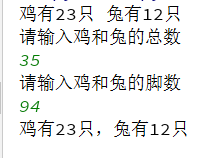# python解决鸡兔同笼问题

# 这个问题，是我国古代著名趣题之一。
# 大约在1500年前，《孙子算经》中就记载了这个有趣的问题。
# 书中是这样叙述的：“今有雉兔同笼，上有三十五头，下有九十四足，
# 问雉兔各几何？这四句话的意思是：
# 有若干只鸡兔同在一个笼子里，从上面数，有35个头
# ；从下面数，有94只脚。求笼中各有几只鸡和兔？
# 列方程式：
# 设鸡有x只，兔有y只
# x+y=35
# 2x+4y=94
# 方法一
a=35
b=94
for x in range(1,a):
y=a-x
if 2*x+4*y==b:
print("鸡有"+str(x)+"只","兔有"+str(y)+"只")

# 方法二
def app(a,b):
x=(4*a-b)/2
if a != 0 and (4*a-b) % (x*2)==0:
y=a-x
if x<0 or y<0:
print("{}只动物{}条腿的情况无解".format(a,b))
else:
print("鸡有{}只，兔有{}只".format(int(x),int(y)))

else:
print("{}只动物{}条腿的情况无解".format(a, b))

a=input("请输入鸡和兔的总数\n")
b=input("请输入鸡和兔的脚数\n")
a= int(a)
b= int(b)
app(a,b)posted @ 2018-12-01 22:35  晴空！  阅读(...)  评论(...编辑  收藏